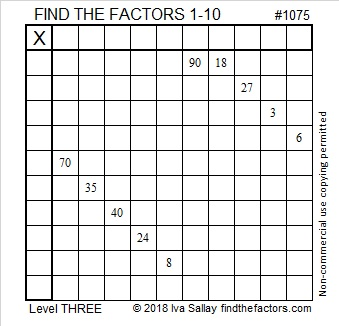# 1075 and Level 3

Begin at the top of this level 3 puzzle and find the factors clue by clue until you reach the bottom. You can solve this puzzle and have fun doing it!Print the puzzles or type the solution in this excel file: 10-factors-1073-1079

Here are some facts about the number 1075:

• 1075 is a composite number.
• Prime factorization: 1075 = 5 × 5 × 43, which can be written 1075 = 5² × 43
• The exponents in the prime factorization are 2 and 1. Adding one to each and multiplying we get (2 + 1)(1 + 1) = 3 × 2  = 6. Therefore 1075 has exactly 6 factors.
• Factors of 1075: 1, 5, 25, 43, 215, 1075
• Factor pairs: 1075 = 1 × 1075, 5 × 215, or 25 × 43
• Taking the factor pair with the largest square number factor, we get √1075 = (√25)(√43) = 5√43 ≈ 32.78719If you had 43 quarters, you would have \$10.75.
If you had 215 nickles, you would also have \$10.75.

1075 is the hypotenuse of two Pythagorean triples:
645-860-1075 which is (3-4-5) times 215
301-1032-1075 which is (7-24-25) times 43

1075 is a palindrome in two consecutive bases:
It’s 898 in BASE 11 because 8(121) + 9(11) + 8(1) = 1075, and
it’s 757 in BASE 12 because 7(144) + 5(12) + 7(1) = 1075

This site uses Akismet to reduce spam. Learn how your comment data is processed.